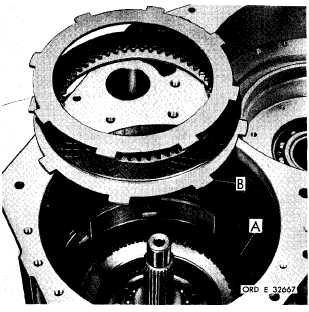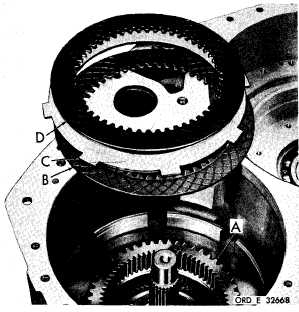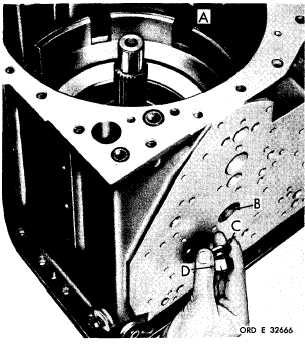Custom SearchP A R   227,   STEPS   7 - 1 0 A S S E M B L Y C H A P   5,   SEC  X X X I II Figure   246   (Step   7) Install   oil   collector   (A)   into   transmission   hous- ing   (B). Figure   247   (Step   8) Install    intermediate-range    clutch    anchor    (A). Install   anchor   bolt   (B).   Install   washer   (C)   and nut   (D).   Tighten   nut   only   finger-tight. Figure   248   (Step   9) Install   two   internal-   (A)   and   two   external-   (B) splined    intermediate-range    clutch    plates,    be- ginning   with   an   internal-splined   plate. Figure   249   (Step   10) I n s t a l l     i n t e r m e d i a t e - r a n g e     r i n g     g e a r     a s s e m - bly   (A).   Install   an   internal-   (B),   an   external- ( C )    a n d    t h e    r e m a i n i n g    i n t e r n a l - s p l i n e d    ( D ), i n t e r m e d i a t e - r a n g e    c l u t c h    p l a t e s. 1 6 5